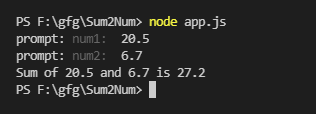# How to add two numbers in console using Node.js ?

• Difficulty Level : Expert
• Last Updated : 26 Oct, 2021

In this article, we will see how to add two numbers in the console using NodeJS. For this purpose, we need to know about a node package called prompt. It helps to take user input from the console. We would use its method prompt.get() to take user input.

Create NodeJS Application: Initialize the NodeJS application using the following command:

`npm init`

Module Installation: Install the prompt module using the following command.

`npm install prompt`

Implementation: Create an app.js file and write down the following code in it.

## app.js

 `// Require would make the prompt ` `// package available to use ` `const prompt = require(``"prompt"``); ` ` `  `// An utility function to add ` `// two numbers ` `function` `add() { ` `  ``// Start the prompt ` `  ``prompt.start(); ` ` `  `  ``// Get two numbers/properties ` `  ``// from user num1 and num2 ` `  ``prompt.get([``"num1"``, ``"num2"``],  ` `  ``function` `(err, res) { ` `    ``// To handle any error if occurred ` `    ``if` `(err) { ` `      ``console.log(err); ` `    ``} ``else` `{ ` `      ``// By default get methods takes ` `      ``// input in string So parseFloat ` `      ``// is used to convert String ` `      ``// into Float ` `      ``var` `sum = parseFloat(res.num1)  ` `        ``+ parseFloat(res.num2); ` ` `  `      ``// Print the sum ` `      ``console.log(``"Sum of "` `+ res.num1  ` `        ``+ ``" and "` `+ res.num2 + ``" is "` `+ sum); ` `    ``} ` `  ``}); ` `} ` ` `  `// Calling add function ` `add();`

Step to run the application: Run the app.js file using the following command.

`node app.js`

Output: Now enter the user input and view output in the console.output

So this is how you can add two numbers in the console using nodeJs. Prompts help us to take input from users. It also supports validation and defaults over the input.

My Personal Notes arrow_drop_up
Recommended Articles
Page :